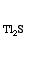Thallium(I) sulfide
Tl2SS 1 Tl 2

Real, long lived, electronically neutral reagent chemical.

Gram formula weight (molecular mass) = 440.83
Melting point = 449 °C
Density = 8 g/cm3
Purity = 99 %
Solubility in water = 1.290e-6 g/100mL
Solubility in organics = insoluble

Toxic
 Wikipedia Page:Thallium(I) sulfide
is defined with respect to the entities below:
The entities below are defined with respect to:
Thallium(I) sulfide
 Ionic material (generic)Mono-bond type material (generic)Type 23 Lewis acid/base complex (generic)chemical compound molecule metal molecular science reaction mechanism ionic material acid base geometry reactivity synthesis science knowledge chemistry Lewis